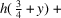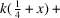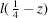International
Tables for
Crystallography
Volume B
Reciprocal space
Edited by U. Shmueli

International Tables for Crystallography (2010). Vol. B, ch. 1.4, p. 122   | 1 | 2 |
https://doi.org/10.1107/97809553602060000761

Appendix A1.4.1. Comments on the preparation and usage of the tables

U. Shmuelia

The straightforward but rather extensive calculations and text processing related to Tables A1.4.3.1through A1.4.3.7and Table A1.4.4.1in Appendices 1.4.3and 1.4.4, respectively, were performed with the aid of a combination of FORTRAN and REDUCE (Hearn, 1973) programs, designed so as to enable the author to produce the table entries directly from a space-group symbol and with a minimum amount of intermediate manual intervention. The first stage of the calculation, the generation of a space group (coordinates of the equivalent positions), was accomplished with the program SPGRGEN, the algorithm of which was described in some detail elsewhere (Shmueli, 1984). A complete list of computer-adapted space-group symbols, processed by SPGRGEN and not given in the latter reference, is presented in Table A1.4.2.1of Appendix A1.4.2.

The generation of the space group is followed by a construction of symbolic expressions for the scalar products; e.g. for position No. (13) in the space group(No. 213, IT I, 1952, IT A, 1983), this scalar product is given by. The construction of the various table entries consists of expanding the sines and cosines of these scalar products, performing the required summations, and simplifying the result where possible. The construction of the scalar products in a FORTRAN program is fairly easy and the extremely tedious trigonometric calculations required by equations (1.4.2.19)and (1.4.2.20)can be readily performed with the aid of one of several available computer-algebraic languages (for a review, see Computers in the New Laboratory – a Nature Survey, 1981); the REDUCE language was employed for the above purpose.

Since the REDUCE programs required for the summations in (1.4.2.19)and (1.4.2.20)for the various space groups were seen to have much in common, it was decided to construct a FORTRAN interface which would process the space-group input and prepare automatically REDUCE programs for the algebraic work. The least straightforward problem encountered during this work was the need to convince' the interface to generate hkl parity assignments which are appropriate to the space-group information input. This was solved for all the crystal families except the hexagonal by setting up a basis' of the form:and representing the translation parts of the scalar products,as sums of such basis functions'. A subsequent construction of an automatic parity routine proved to be easy and the interface could thus produce any number of REDUCE programs for the summations in (1.4.2.19)and (1.4.2.20)using a list of space-group symbols as the sole input. These included trigonal and hexagonal space groups with translation components of. This approach seemed to be too awkward for some space groups containing threefold and sixfold screw axes, and these were treated individually.

There is little to say about the REDUCE programs, except that the output they generate is at the same level of trigonometric complexity as the expressions for A and B appearing in Volume I (IT I, 1952). This could have been improved by making use of the pattern-matching capabilities that are incorporated in REDUCE, but it was found more convenient to construct a FORTRAN interpreter which would detect in the REDUCE output the basic building blocks of the trigonometric structure factors (see Section 1.4.3.3) and perform the required transformations.

Tables A1.4.3.1–A1.4.3.7were thus constructed with the aid of a chain composed of (i) a space-group generating routine, (ii) a FORTRAN interface, which processes the space-group input and writes' a complete REDUCE program, (iii) execution of the REDUCE program and (iv) a FORTRAN interpreter of the REDUCE output in terms of the abbreviated symbols to be used in the tables. The computation was at a `one-group-at-a-time' basis and the automation of its repetition was performed by means of procedural constructs at the operating-system level. The construction of Table A1.4.4.1involved only the preliminary stage of the processing of the space-group information by the FORTRAN interface. All the computations were carried out on a Cyber 170–855 at the Tel Aviv University Computation Center.

It is of some importance to comment on the recommended usage of the tables included in this chapter in automatic computations. If, for example, we wish to compute the expression:, use can be made of the facility provided by most versions of FORTRAN of transferring subprogram names as parameters of a FUNCTION. We thus need only two FUNCTIONs for any calculation of A and B for a cubic space group, one FUNCTION for the block of even permutations of x, y and z:where TPH, TPK and TPL denote,and, respectively, and a similar FUNCTION, say O(P,Q,R), for the block of odd permutations of x, y and z. The calling statement in the calling (sub)program can thus be:A small number of such FUNCTIONs suffices for all the space-group-specific computations that involve trigonometric structure factors.

References

Computers in the New Laboratory – a Nature Survey (1981). Nature (London), 290, 193–200.
International Tables for Crystallography (1983). Vol. A, Space-Group Symmetry, edited by Th. Hahn. Dordrecht: Reidel.
International Tables for X-ray Crystallography (1952). Vol. I, Symmetry Groups, edited by N. F. M. Henry & K. Lonsdale. Birmingham: Kynoch Press.
Hearn, A. C. (1973). REDUCE 2.0 user's manual. University of Utah, Salt Lake City, Utah, USA.
Shmueli, U. (1984). Space-group algorithms. I. The space group and its symmetry elements. Acta Cryst. A40, 559–567.